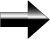Example 1

 Linear equation Steps to solve equation The math behind the solution x + 4 = 1 The first step is to get "x" on one side of  the equation by subtracting 4 from both sides: x + 4 - 4 = 1 - 4 x = -3

Example 2

 Linear equation Steps to solve The math behind the solution 3x - 5 = 10 The first step is to get "x" to one side of the equation by adding 5 to both sides: 3x - 5 + 5 = 10 + 5 3x = 15 The second step is to divide both sides by 3 to get x by itself: 3x / 3 = 15 / 3 x = 5 Check your work with the original equation: (3 * 5) - 5 = 10 15 - 5 = 10      10 = 10

Example 3 (using negative numbers

 Linear equation Steps to solve The math behind the solution 3x + 5 = -10 The first step is to get "x" to one side of the equation by subtracting 5 from both sides: 3x + 5 - 5 = -10 - 5 3x = -15 Second step is to divide both sides by 3 to get x by itself: 3x / 3 = -15 / 3 x = -5 Check your work with the original equation: (3 * -5) + 5 = -10 -15 + 5 = -10      -10 = -10

Example 4 (two variables)

 Linear equation Steps to solve The math behind the solution 2x - 2y = 4 The first step is to get "x" on one side of the equation by adding 2y to both sides: 2x - 2y + 2y = 4 + 2y 2x = 4 + 2y Second step is to divide both sides by 2 to get x by itself: 2x / 2 = (4 + 2y) / 2 x = 2 + y Check your work with the original equation: 2 * (2 + y) - 2y = 4 4 + 2y - 2y = 4 4= 4

Main Pre-Calculus PageLinear Equations